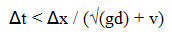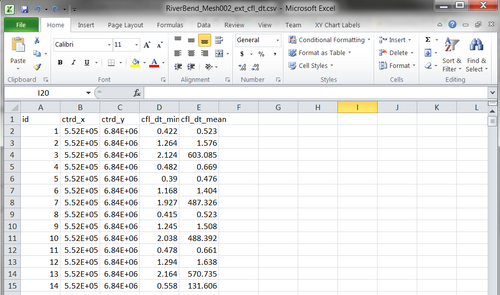# Introduction

The computational effort required to run a model simulation is a function of:

• The timestep, which in turn is limited by the element in the model domain that limits the Courant-Frederic-Lewy (CFL) number.
• The number of active, wet elements (or cells) in the model domain (note that this can vary from one timestep to another).
• The spatial extent of a TUFLOW FV model (ie the area to be modelled).
• The temporal extent of a TUFLOW FV model (ie the duration of model simulations) is typically guided by the temporal extent of the key physical processes to be represented. * How complex the numerical processes are (eg. A second order hydrodynamic simulation requires additional computational effort compared to a first order simulation).

Additionally, the speed of a simulation is influenced by the power of the computer used to do the modelling. However, before considering buying a new more powerful computer, users should first consider the following items.

# Model Timestep

TUFLOW FV uses an adaptive timestep which is calculated throughout a simulation based on Courant-Frederic-Lewy (CFL) criteria. The Courant number limits each timestep in a model simulation as follows:

```t = timestep, x = nominal cell length, g = gravity, d = water depth, v = velocity
```

Based on this criteria, a models timestep is dependent upon the single element within a model that has the highest Courant number.

Understanding this concept is important. This means that a small element in deep water and/or with a high velocity will likely become the limit for the timestep and hence the overall simulation time. Reviewing which element is responsible for limiting the CFL condition is an important step in designing an efficient model mesh.

Is the limiting element in your area of interest? Does your mesh design intentionally require small cells that location, or is it an unintended error in mesh? Sometimes, increasing the sizes of a handful of CFL limiting cells can increase the model timestep significantly, hence making a more efficient model.

## Timestep Review

The calculated model timestep is reported in a variety of locations, including the:

1) Simulation DOS window or simulation log file (<simulation name>.log)

2) cfl dt log file (<simulation name>_ext_cfl_dt.csv): A summary log reporting the minimum and mean timestep calculated for each element face within the model.Excel can be used to sort the cfl dt log data using the following steps:
a) Open the cfl dt log file (<simulation name>_ext_cfl_dt.csv) in Excel
b) Highlight the entire dataset
c) Select Data > Sort
d) Sort by, Column: cfl_dt_min , Sort On: Values , Order : Smallest to Largest

Completing these steps on a sample dataset identifies four element faces which have a timestep limit of 0.06 seconds. The next smallest timestep limit is 0.26 seconds.

With this knowledge, we now need to locate where these elements are located within our model mesh. Then we can determin if they are required at their current resolution, or alternatively are an unintended mistake resulting from the mesh creation process. Removing or increasing the resolution of these elements will significantly improve our models runtime efficiency (i.e. decreasing the simulation runtime).

## Limiting Timestep Location Identification

The external cfl timestep file can be viewed in SMS. The following link works through the steps required to load the dataset into SMS: | TUFLOW FV Mesh Performance Mapping in SMS

Highlighted in blue within the above figure, the timestep limit of 0.06 seconds is occurring at the face of two small triangular elements. These timestep limiting elements are not intentionally so refined, they are an error in the mesh generation process. Updating the mesh, to remove these two small timestep limiting elements increases the minimum simulation timestep from 0.06 seconds to 0.26 seconds. As such, updating two cells in the mesh has increased the efficiency by a factor four (decreasing the simulation runtime from 22 minutes to 5 minutes).

# Model Resolution

The number of active, wet elements (or cells) in the model domain influences the computational effort required to run a model simulation. Doubling a models resolution results in up to an 8 fold increase in simulation time (4 times more cells and ½ the timestep).

The flexible mesh consists of a network of irregular triangular and quadrilateral elements. This has inherent advantages, including:

• Mesh resolution can be adjusted according to the needs of the study (i.e. fine resolution in the area of interest, coarser resolution in the regional extents). Thus, a range of spatial scales can be modelled without requiring nesting.
• Mesh alignment can fit bathymetric contours and boundary extents, optimising mesh resolution. This is particularly relevant in regions with complex bathymetric features.
• Specific features, such as man-made developments, infrastructure can be included in the model mesh precisely.

To exploit these advantages, the mesh needs to be designed carefully and appropriately for the specific model application. Over the life cycle of a modelling project, a well assembled mesh will save time (both the modellers and the computers).

# Model Scheme

TUFLOW FV can be run in various hydrodynamic modes, including:

• First Order Two Dimensional Hydrodynamic
• Secord Order Two Dimensional Hydrodynamic
• Three Dimensional Hydrodynamic

The 'First Order Two Dimensional Hydrodynamic' scheme is the most basic mode, requiring the least computation effort. This scheme is sufficient in the vast majority of flood/coastal applications. Modelling using the first order scheme is recommend to determine its adequacy prior to considering the more computationally intensive 'Second Order' or 'Three Dimensional' options.

## First Order or Second Order

Higher order spatial schemes will produce more accurate results in the vicinity of sharp gradients due to reduced numerical diffusion, however they will be more prone to developing instabilities and are more computationally expensive. The first-order schemes assume a piecewise constant value of the modelled variables in each cell, whereas the second-order schemes perform a linear reconstruction.

As a general rule of thumb, initial model development should be undertaken using low-order schemes, with higher-order spatial schemes tested during the latter stages of development. If a significant difference is observed between low-order and high-order results then the high-order solution is probably necessary, or alternatively further mesh refinement is required.

## 2D or 3D

Three-dimensional simulations can be performed within TUFLOW FV using either sigma-coordinate or a hybrid z-coordinate vertical mesh. Three-dimensional simulations can optionally use a mode-splitting approach to efficiently solve the external (free-surface) mode in 2D at a timestep constrained by the surface wave speed while the internal 3D mode is updated less frequently.

As a step in the development of a 3D model a 2D simulation should be performed first. Once the 2D model has been optimised and output verified the modeller may then choose to perform a 3D simulation.

# Model Nesting

A TUFLOW FV model can be nested. This is advantageous when model simulations are becoming excessively long, or when a regional model is performed over a long period and local models are run for sub-periods from it. In such circumstances, select specific model outputs at sufficient resolution from which boundary conditions for the nested models can be extracted.

# Parallel Processing

TUFLOW FV is parallelised for multi-processor machines using the OpenMP implementation of shared memory parallelism. This means that a TUFLOW FV model simulation will run faster if there is more than one processor (or thread) on a single computer. The increase in computational speed is not quite linear with the number of threads.

Unless the user decides otherwise, TUFLOW FV will run using the maximum number of threads available to it (limited by the licence). This means that, by default, TUFLOW FV will run as fast as the host computer permits it to.